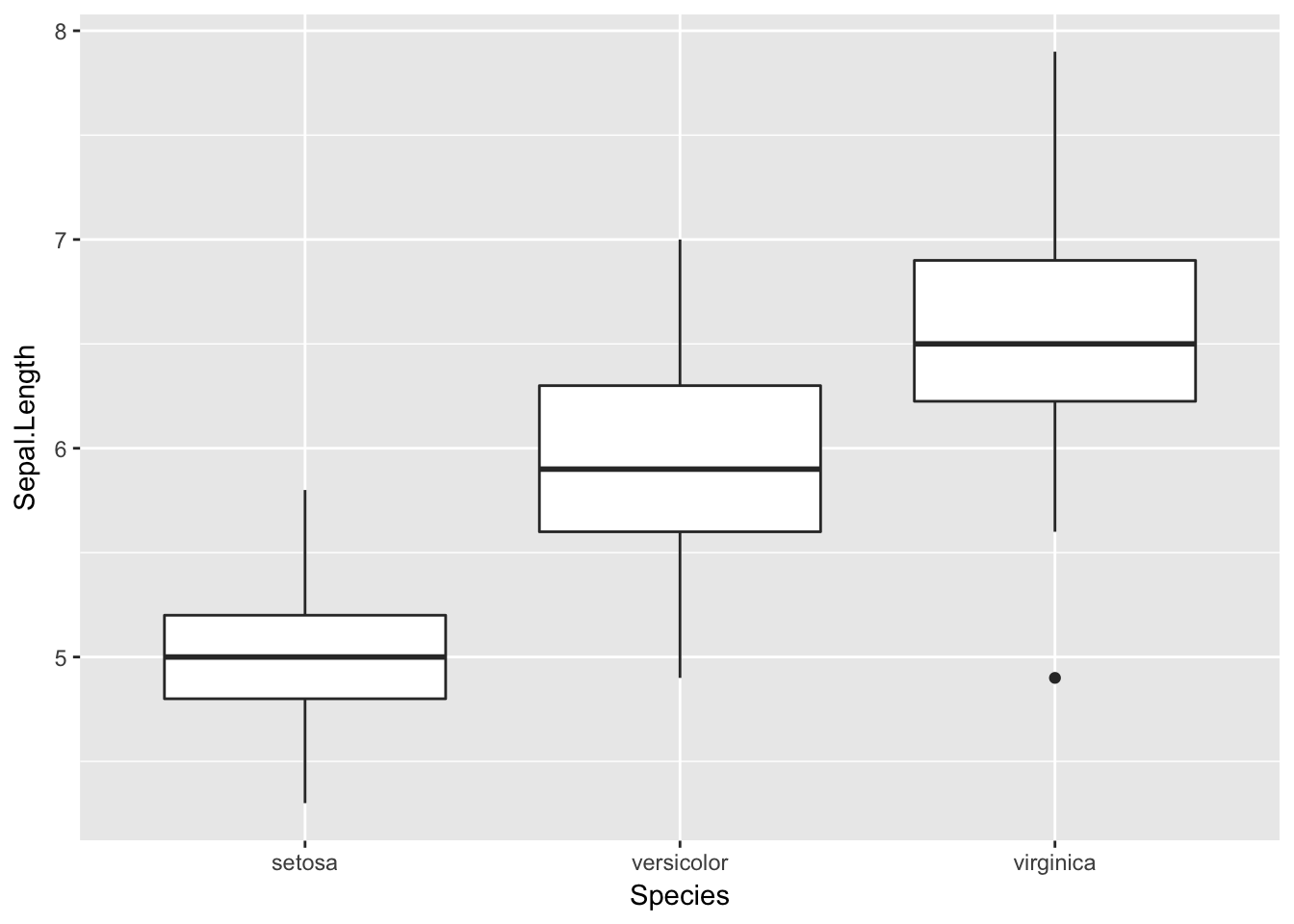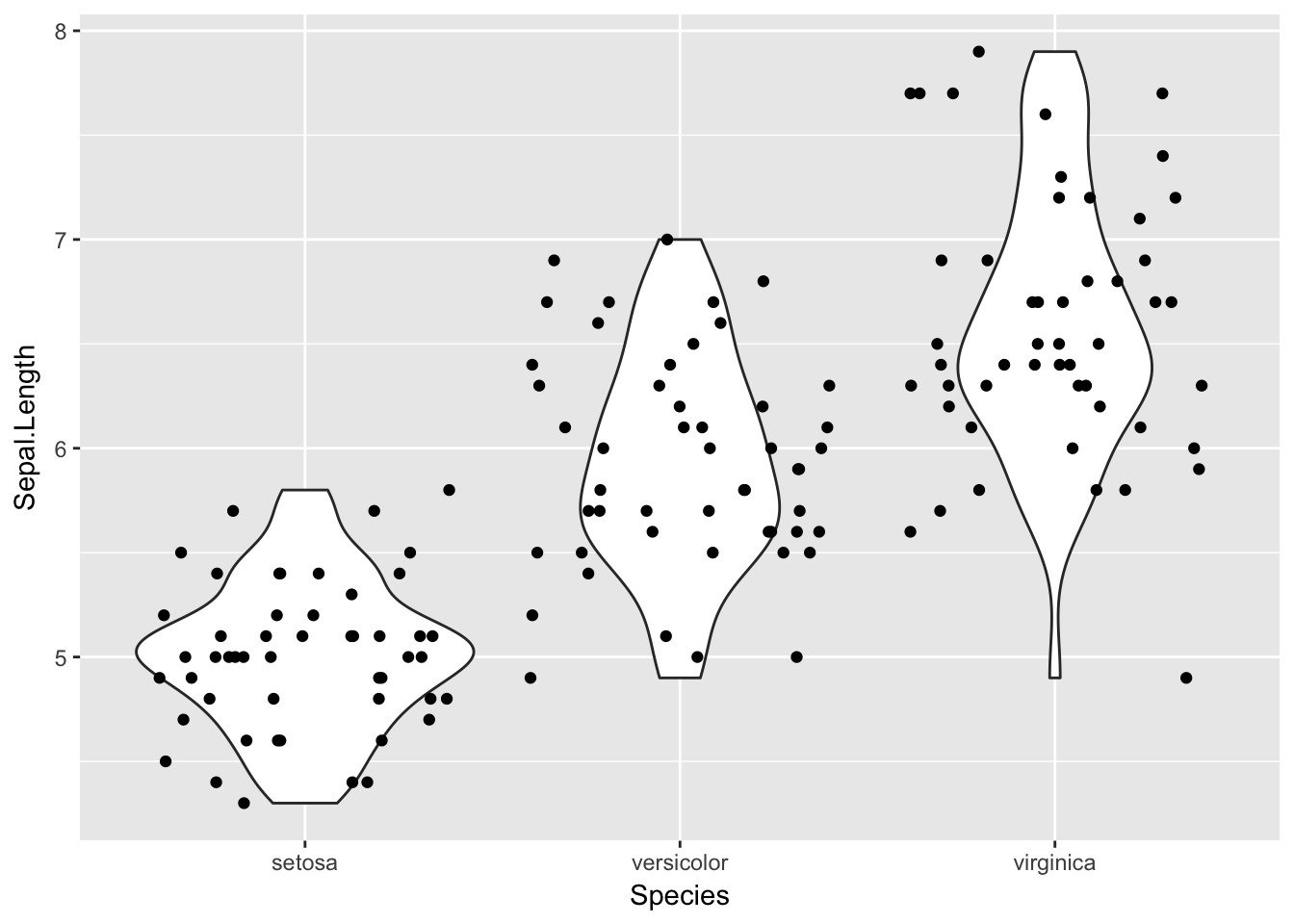# First we will need to set up your working directory

1. Set up working director:
• Click Session - Choose Directory -> Desktop -> RNanoCourse -> rscripting_workshop

The basics of a function are: FunctionName <- function(args1,args2, …) { statements return (something) }

1. Writing a simple function. Paste the following code in your console.
``````SumOfNumbers <- function(x,y) {
z = x + y
return (z)
}``````

This is a a simple function called SumOfNumbers, which takes two arguments x and y and returns their sum.

1. Use the function SumOfNumbers to add x=35 and y=43?

2. Create a function SumOfSquares which takes two arguments x and y and returns the sum of the squares

3. Functions can return a list of multiple arguments for more complex calculations, using the list() function

``````MultipleCalculations <- function(x,y) {
z = x + y
c = x^2 + y^2
a = list(result1=z, result2=c)
return (a)
}``````
1. Try the function on MultipleCalculations on x=5 and y=4? What does it return

2. Functions can take a variety of argument types
• single numbers
• vectors
• matrices

Make the vectors v1 and v2 and use the function SumOfNumbers to add the two. What is the result?

``````v1 <- c(1,4,5)
v2 <- c(-1,20,3)
SumOfNumbers(v1,v2)``````
``##   0 24  8``

Make the matrices m1 and m2 and use the function SumOfSquares to get the sum of squares the two. What is the result?

``````m1 <- matrix( c(4, 3, -3, 1, -3, 4), ncol=2)
m2 <- matrix( c(-2, 2, -2, 0 , 5, 2), ncol=2)``````

### Summary:

• Check for errors along the way.
• Try out your function with simple examples to make sure it’s working properly

# Loops

We have written function that perform a single operation. But we want to write a function that can be used many times to do the same operation on lots of different data.

• For: Allows operations to be executed repeatedly
• While: Allows operations to be executed repeatedly until a condition is meet
• Repeat: Allows operations to be executed repeatedly until a a user defined condition is meet

## For Loops

The for loop is used when iterating through a list.

1. A simple for loop that prints out numbers 1 through 4:
``````for(i in 1:4) {
print(i)
}``````
``````##  1
##  2
##  3
##  4``````
1. Create a matrix and find the sum of each row.
``````example.matrix <- matrix( c(4, 8, 31, 1, 0, 20, 10, 20, 15,12, 34, 2), ncol=4)

MatrixRowSum <- function(m){
vector.row <- matrix()
for (i in 1:nrow(m)){
row.sum <- 0
for (j in 1:ncol(m)){
row.sum <- row.sum + m[i,j]
}
vector.row[i] <- row.sum
}
return(vector.row)
}

MatrixRowSum(example.matrix)``````
``##  27 62 68``
1. Write the a function, MatrixRowMean to find the mean of each row for example.matrix.

## Apply functions

There are several function in R that allow you to apply some function to a series of objects (eg. vectors, matrices, dataframes or files). They include:

• apply : Applies a function to every column or row of an array and returns the results in an array.
• lapply: Applies a function to elements in a list or a vector and returns the results in a list.
• sapply: Applies a function to elements in a list and returns the results in a vector, matrix or a list.
• tapply: Applies a function to each cell of an array.

apply(X, MARGIN, FUN, …)

• X : Array or Matrix
• Margin: Specify which margin we want to apply the function to and which margin we wish to keep. If the array we are using is a matrix then we can specify the margin to be either 1 (apply the function to the rows) or 2 (apply the function to the columns).
• FUN: Can be any function that is built in or user defined to be applied to X
• …: Additional arguments for that will be passed to the function.
1. Example of finding the sum of each row using the apply function.
``apply(example.matrix,1,sum)``
``##  27 62 68``
1. Find the mean and the median of each row using the apply function.

# Writing Scripts

An R script is simply a text file containing the same commands that you would enter on the command line of R.

## Plotting

### Boxplots

A standard way of showing the distribution of the data.

1. Load iris sample data and install necessary packages. Make a boxplot of Sepal.Length by Species.
``ggplot(data = iris, aes(Species, Sepal.Length)) + geom_boxplot()``### Violin plots

A violin plot is more informative than a plain box plot. In fact while a box plot only shows summary statistics such as mean/median and interquartile ranges, the violin plot shows the full distribution of the data.

1. Make a violin plot of Sepal.Length by Species.
``ggplot(data = iris, aes(Species, Sepal.Length)) +  geom_violin() + geom_jitter(height = 0)``Can we generalize a function to make both boxplot and violin plot side by side?

Open multi_plots.R and lets walk through the function.

1. Plot Sepal.Length and Species using the MultiPlots function.
``````source('multi_plots.R')
MultiPlots(iris, aes(Species, Sepal.Length), "Boxplot and Violin Plot")``````